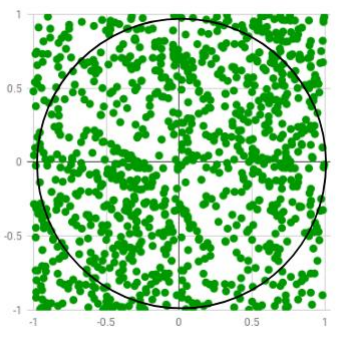# CSI6110 Software Development Processes Editing Services### CSI6110 Software Development Processes Editing Services

This assignment will explain about different forms of software development processes, including sequential, iterative and formal models as well as those approaches based on reusability. In addition, it covers method measurement and software estimation techniques, the use of software metrics and automated tool support.

Assessment 2

This assessment has 35% value

Learning outcomes

This assignment has following learning outcomes:

1. Apply techniques to estimate software project costs, duration and effort

2. Identify software capability determination and evaluation techniques

3. Reflect on the need for measurement and software metric formulation and evaluation

Background

The Personal Software Process (PSP) attempts to focus on improving individual performance in the upper levels of the CMM. The PSP recommends establishing metrics to measure aspects of the software process and to test personal competency by writing programs measured with these metrics.Students are required to create two small programs in Python. You need to analyze the time taking to write the program. Also, you need to record two things:

1. How it takes you to write the program

2. Defects involved in it as part of the development.

1. Program size i.e. how many lines of code you think you will write

2. Time to complete i.e. the time it will take you to write the program in hours or days, including time for defect detection/removal

Note- It is essential that the estimates are calculated before you start writing your programs.

This is an individual assignment with 5% value. Students have to write a Python program to count (in LOC) the total program size of a Python program and print out a count of the LOC. Consider whether a comment counts as a “line”. Justify your answer.

This is an individual assignment with15% value. Students have to read the details on the programming task, the tests required and some background on the technique used. You can use numerical methods to approximate the area under a curve.

The graph shows of r 2 = x2 + y 2 (i.e., a circle). The Monte Carlo method can be used to approximate the value of π. It also shows a circle of radius 1 (so r =1 in the above equation), enclosed by a 1 × 1 square. The area of the circle is πr2=π/4, the area of the square is 1. Dividing the area of the circle by the area of the square, the result is π/4. Area of circle/Area of square = πr2 /4r2 = π/4 A way to calculate π is to generate a large number of uniformly distributed random points between (0, 0) and (1, 1), these points would then be at any position within the square.

You must keep track of the total number of points and the number of points that are inside the circle. By dividing the number of points within the circle, (call this Ninner) by the total number of points, (call this Ntotal), this calculates a value that is an approximation of the ratio of the areas calculated above or π/4.

Now, Write a Python program that calculates the value of π for various numbers of iterations (random points). How much iteration (points) do you need to get a correct value of π to 2, 4 and 6 decimal places? How did you determine the value of π to 6 decimal places correctly? How do you know it is correct? Consider the relative error of your approximation, given by error = (πapprox. – π)/ π. Graph this value for various iterations. When should you stop iterating?This is a group assignment with 10% value. Students are required to pool your values for time to complete. There are different predictive models like COCOMO in general form:

Person-Months = a*KLOC^b where “a” and “b” are defined constants and “*” and “^” are the usual multiplication and exponentiation operators. When using this type of model, two questions arise. First, where do these constants come from and second, could this model be used as-is or would it need to be calibrated for each organization?

The constants come from fitting to known data about projects (their KLOC and PM results). Calibration is essential as the type of projects that were used to derive the original values for the modes of COCOMO are not necessarily in the same domain as the software development firm which wishes to use the model. A common method used to fit curves to data is least-squares regression.

For a linear model, the equation is often expressed as:

y = β1*x + β0, where “β1” is the slope of the line and“β0”is the point where the line crosses they-axis (called the y-intercept).

Students are required to calculate constants for a predictive model, based on your pooled data. The COCOMO equation will need to be transformed into a linear equation to use the linear model above.

Log Person-days = Log a + b * Log LOC

There are several tools/packages/languages you could use to perform this task. The line of best fit calculation (and graph) will be the same for each member of your group. Include the names of your group members in this task.